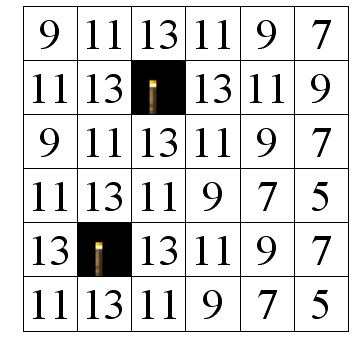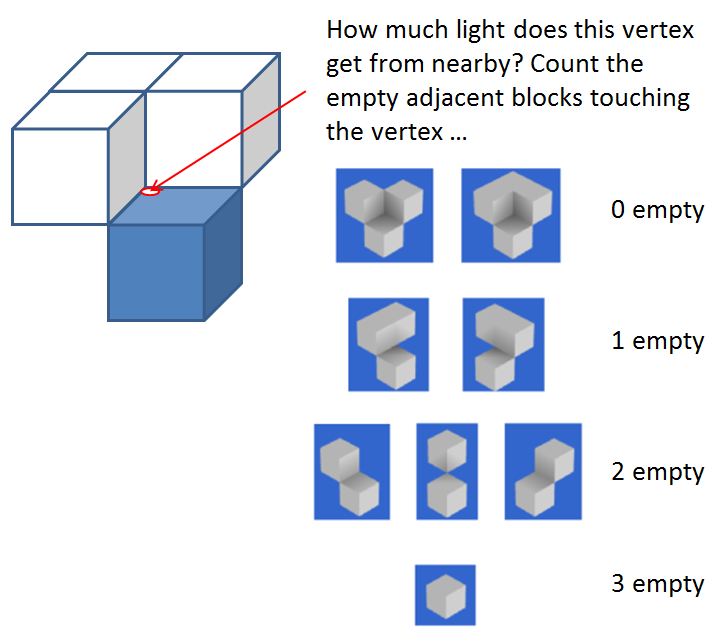## Sky Light and Block Light

The basic lighting model in Minecraft is made up of two components; Sky Light and Block Light.  Each block in the world has a value between 0 - 15 for Sky Light and also a value between 0 - 15 for Block Light.
1. Sky Light is the light that this block is receiving from the sky, either directly or indirectly.
2. Block Light is the light that this block is receiving from other light sources nearby (torches, glowstone, etc).
See here for some more background information.

World.computeLightValue() is used to calculate the Sky Light and Block Light for each block.  The basic concepts are:
1. Each block has an Opacity value ( .getLightOpacity() ), which determines how much light it receives from its six neighbours.  A low opacity (eg 1) means the light level decreases by 1 from its brightest neighbour, higher opacity (eg 3) leads to a correspondingly larger decrease.  This calculation is performed separately for both the Sky Light and the Block Light.
2. Some blocks emit light (eg glowstone) - if this is more than the Block Light coming from its neighbours, the Block Light is set to this emitted value.
3. If the block has only open sky or transparent blocks above it, its Sky Light is 15.Two-dimensional example for propagation of Block Light, with opacity = 2.  Sky Light is propagated in the same way.
When the block is rendered, the Block Light and Sky Light values for the block are used to set the brightness.  This is done as follows:
1. The Block Light and Sky Light values are combined into a single 32-bit integer, for example by Block.getMixedBrightnessForBlock().  The upper 16 bits contain the Sky Light multiplied by 16, and the lower 16 bits contain the Block Light multiplied by 16.
2.  tessellator.setBrightness() is called with this combined "mixed brightness" value, which is interpreted by the Tessellator as two 16 bit coordinates (i.e. [BlockLight, SkyLight]) for a texture called the lightMap texture (GL_TEXTURE1) - see picture below.
3.  When OpenGL renders the block face, it first draws the icon texture (GL_TEXTURE0) as previously described then "modulates" it using the appropriate texel from the lightMap, i.e. as given by the [BlockLight, SkyLight] coordinate.  (This process is called Multitexturing).The lightMap texture (GL_TEXTURE1), which has 16x16 texels .  Each texel corresponds to a combination of Block Light and Sky Light.
Why does Minecraft bother to do it this way?  There are at least two good reasons
1. Treating Block Light and Sky Light separately allows more realistic lighting effects.  Looking at the lightMap texture you can see that Block light imparts a reddish/brownish colour whereas Sky Light does not.  This simulates the yellowy light produced by (eg) a torch.
2. The lightMap can be updated very easily to change the lighting of the entire scene- for example depending on the time of day (eg full sun, sunset); to create the effect of a flickering torch; or when the player drinks a night vision potion.  This updating is performed by EntityRenderer.updateLightMap().

## Face-dependent lighting effects

In addition to the per-block lighting described above, Minecraft can also perform some extra lighting calculations for each block face to increase the realism of the lighting.  These are performed in one of the following methods:

### .renderStandardBlockWithColorMultiplier()

This method renders the six faces with a different lighting intensity depending on which direction the faces points:
1. Top face (Ypos) is at full intensity
2. Bottom face (Yneg) is 50% intensity
3. Z-side faces (Zpos, Zneg = South, North) are 80% intensity
4. X-side faces (Xpos, Xneg = East, West) are 60% intensity.
This lighting is applied to each face using Tessellator.setColorOpaque() ;  when the texture is rendered it is multiplied by the "colour" value (in this case a shade of grey).

In some cases an RGB colour multiplier is also passed in by the caller (for example underwater gives blocks a blue tint) which is multiplied by the % intensity described above.

The lighting of the face is also affected by the [BlockLight, SkyLight] mixed-brightness in the usual way.  The diagram below summarises these steps.Schematic showing how Minecraft applies lighting effects: (1a) face-dependent intensity and (1b) colour multiplier, then (2) [Blocklight,Skylight] lightmap

### .renderBlockWithAmbientOcclusion(), .renderStandardBlockWithAmbientOcclusion()

Ambient Occlusion is a method for calculating the amount of light shining on a surface based on nearby blocks.  A brief introduction on the approach used by minecraft is here.   Briefly, for each vertex, an average light intensity is calculated from the three adjacent blocks plus the block which touches the face.  In the example below for the red circled vertex this is the three blocks in white plus the block resting on top of the face (not shown).  If all these four blocks are transparent, the "Ambient Occlusion" light intensity will be 1.0.  Each opaque block reduces light received by the vertex - if all four blocks are opaque the intensity will be 0.2.  This "Ambient Occlusion" value is then multiplied by the colour multiplier and used to .setColorOpaque() for that vertex.
The Mixed Brightness for the vertex is calculated as the average of these four adjacent blocks.
(There are some extra details about whether the corner block is included or not, but you get the basic idea).
OpenGL applies smoothing when drawing the face, so that the lighting effect is graded smoothly over the face between the vertices.Ambient Occlusion algorithm.  Diagram adapted from 0fps.

Which method does minecraft choose?
If Smooth lighting is on and the block doesn't glow (i.e. Block.lightValue == 0):  Ambient Occlusion.
Otherwise:  .renderStandardBlockWithColorMultiplier()